##Hands-On-Physics

### Advanced Mechanics Concepts: GLOSSARYAcceleration is the rate at which the velocity vector changesCharges are the basis of electricity. A charge exert forces on other charges. The smallest charge is the charge of an electron, 0.000000000000000016 Coulombs.Current is the rate of flow of electrical charge.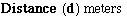One of the undefined qualities of physics, it measures the separation of two points.

Dependent Variable
When two variables are related, we say that one depends on the other. This variable is called the dependent variable. The"other" variable is free to roam so it is called the independent variable. In a scientific experiment, the experimenter chooses values for the independent variable, runs the experiment, and measures the dependent variable. Ordered pairs of choosen and measured values (independent and dependent) are often plotted on a two dimensional graph for visualization. The dependent variable is traditionally plotted on the vertical axis.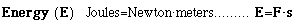The energy of an object increases when work is done on it. An increase in energy may heat up the object, speed it up, lift it up, or all of the above.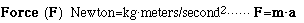The strength of a force is defined by the rate at which it can speed up one kilogram of mass.

Independent variable
When two variables are related, one of the variable is free to roam so it is called the independent variable. The other variable depends on the first, so it gets the name dependent. In a scientific experiment, the experimenter chooses values for the independent variable, runs the experiment, and then measures the dependent variable. Ordered pairs of choosen and measured values (independent and dependent) are often plotted on a two dimensional graph for visualization. The independent variable is traditionally plotted on the horizontal axis.Notice that this energy of motion is proportional to the square of the speed. The unit of Joule may also be expressed as kg(m/sec)(m/sec).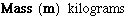One of the undefined qualities of physics, mass is the measure of inertia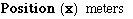The position of an object is its distance from zero. The position depends on the zero (origin) of the measurement.Power is the rate of working. When power is transmitted electrically, the amount is the product of voltage (V) with current (I).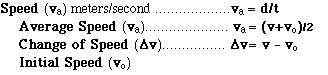Speed is simply the rate of position change, but for analysis and problem solving speed has several faces. The speed at an instant is can be approximated with an average speed measured over a short time interval. Initial speed is the speed of an object at the beginning of a measurement, an intial condition. The average speed is the ratio of distance covered to the time it takes, or the average of the final and intial speeds. The change in speed is the difference between final and inital speeds. The symbol v is used for speed because speed is the size of the velocity vector.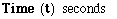Time is one of the undefined qualities of physics. We all know what it is and agree to use a second as one unit of time.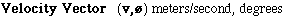The "length" of the velocity vector is the speed. The direction of the motion (ø), is also part of the velocity vector.Voltage is an energy measure, the energy carried by one coulomb of electrical charge. The voltage between two points in a circuit is the amount of energy available for pushing each coulomb of charge from one of these points to the other.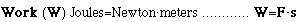Work is done on an object whenever it moves because it is pushed or pulled. Work increases the energy of the object.